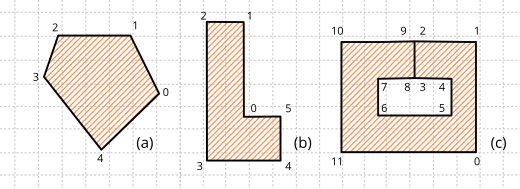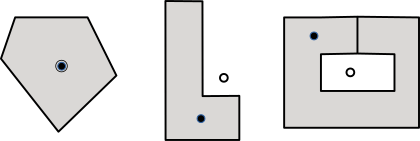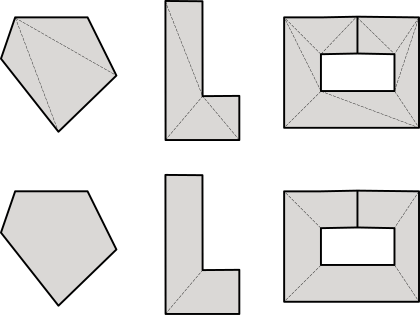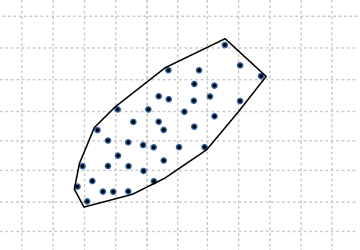# 2D Polygons

This page has been automatically translated using the Google Translate API services. We are working on improving texts. Thank you for your understanding and patience.

## Functions

 Pol2D* pol2d_create (...) Pol2D* pol2d_convex_hull (...) Pol2D* pol2d_copy (...) void pol2d_destroy (...) void pol2d_transform (...) const V2D* pol2d_points (...) uint32_t pol2d_n (...) real pol2d_area (...) Box2D pol2d_box (...) bool_t pol2d_ccw (...) bool_t pol2d_convex (...) V2D pol2d_centroid (...) V2D pol2d_visual_center (...) ArrSt(Tri2Df)* pol2d_triangles (...) ArrSt(Pol2Df)* pol2d_convex_partition (...)

Polygons are widely versatile figures, since they allow us to define arbitrary regions delimited by rectilinear segments. Geom2D supports so-called simple polygons, which are those whose sides cannot intersect each other.

We can classify the polygons into three large groups (Figure 1):

• Convex: The most "desired" from the point of view of calculation simplicity. They are those where any segment that joins two interior points, is totally within the polygon.
• Concave: Or not convex. The opposite of the above. It is one that has an interior angle of more than 180 degrees.
• Weakly: It is one that presents holes through "cut" segments where two vertices are duplicated to allow access and return of each hole. It is an easy way to empty the interior of regions without requiring multiple cycles. The calculation of areas and collisions will take into account these cavities.
•Figure 1: 2D polygons. (a) Convex, (b) Concave, (c) Weak. All of them defined counter-clockwise.

## 1. Polygon center

It is difficult to define a central point in a figure as irregular as a polygon can be. Normally we will interpret as such the centroid or center of mass but, in non-convex cases, this point can be located outside the polygon. In labeling tasks, it is necessary to have a representative point that is within the figure. We consider the visual center to be that point within the polygon located at a maximum distance from any edge (Figure 2). In convex polygons it will coincide with the centroid.

• Use pol2d_centroidf to get the centroid of the polygon.
• Use pol2d_visual_centerf to get the visual center of the polygon. It implements an adaptation of the polylabel algorithm of the MapBox project.
•Figure 2: "Central" point of a polygon. Line: Centroid, Fill: Visual or Label Center.

## 2. Polygon decomposition

Certain calculations or rendering tasks can be considerably optimized if we reduce the complexity of the geometry to be treated. Decomposing a polygon is nothing more than obtaining a list of simpler polygons whose union is equivalent to the original figure (Figure 3). As an inverse operation, we would have the calculation of the convex hull, which is obtaining the convex polygon that encloses a set of arbitrary points (Figure 4).

• Use pol2d_trianglesf to get a list of the triangles that make up the polygon.
• Use pol2d_convex_partitionf to get a list of convex polygons equivalent to the polygon.
• Use pol2d_convex_hullf to create a convex polygon that "wraps" a set of points.
•Figure 3: Decomposition of a polygon by triangulation or convex components.Figure 4: Convex hull of a set of points.

## pol2d_create ()

Create a new polygon.

```Pol2Df*
pol2d_createf(const V2Df *points,
const uint32_t n);

Pol2Dd*
pol2d_created(const V2Dd *points,
const uint32_t n);

Pol2D*
Pol2D::create(const V2D *points,
const uint32_t n);
```
 points List of points that make up the polygon. n Number of points.

Return

The polygon created.

## pol2d_convex_hull ()

Creates the minimum convex polygon that surrounds a set of points (Convex Hull).

```Pol2Df*
pol2d_convex_hullf(const V2Df *points,
const uint32_t n);

Pol2Dd*
pol2d_convex_hulld(const V2Dd *points,
const uint32_t n);

Pol2D*
Pol2D::convex_hull(const V2D *points,
const uint32_t n);
```
 points Points list. n Number of points.

Return

The polygon.

## pol2d_copy ()

Create a copy of the polygon.

```Pol2Df*
pol2d_copyf(const Pol2Df *pol);

Pol2Dd*
pol2d_copyd(const Pol2Dd *pol);

Pol2D*
Pol2D::copy(const Pol2D *pol);
```
 pol The original polygon.

Return

The copy.

## pol2d_destroy ()

Destroy the polygon.

```void
pol2d_destroyf(Pol2Df **pol);

void
pol2d_destroyd(Pol2Dd **pol);

void
Pol2D::destroy(Pol2D **pol);
```
 pol The polygon. Will be set to `NULL` after destruction.

## pol2d_transform ()

Apply a 2D transformation.

```void
pol2d_transformf(Pol2Df *pol,
const T2Df *t2d);

void
pol2d_transformd(Pol2Dd *pol,
const T2Dd *t2d);

void
Pol2D::transform(Pol2D *pol,
const T2D *t2d);
```
 pol The polygon. t2d 2D transformation.

Remarks

The polygon does not save the original coordinates. Successive transformations will accumulate.

## pol2d_points ()

Gets the vertices that make up the polygon.

```const V2Df*
pol2d_pointsf(const Pol2Df *pol);

const V2Dd*
pol2d_pointsd(const Pol2Dd *pol);

const V2D*
Pol2D::points(const Pol2D *pol);
```
 pol The polygon.

Return

Pointer to an array of vertices.

Remarks

Do not modify the returned array. Copy if necessary.

## pol2d_n ()

Gets the number of vertices that make up the polygon.

```uint32_t
pol2d_nf(const Pol2Df *pol);

uint32_t
pol2d_nd(const Pol2Dd *pol);

uint32_t
Pol2D::n(const Pol2D *pol);
```
 pol The polygon.

Return

The number of vertices.

Remarks

It is the same value as the one used in the constructor pol2d_createf.

## pol2d_area ()

Gets the area of ​​the polygon.

```real32_t
pol2d_areaf(const Pol2Df *pol);

real64_t

real
Pol2D::area(const Pol2D *pol);
```
 pol The polygon.

Return

The area.

## pol2d_box ()

Gets the geometric limits of the polygon.

```Box2Df
pol2d_boxf(const Pol2Df *pol);

Box2Dd
pol2d_boxd(const Pol2Dd *pol);

Box2D
Pol2D::box(const Pol2D *pol);
```
 pol The polygon.

Return

Box aligned with the axes, defined by the minimum and maximum vectors.

## pol2d_ccw ()

Gets the winding order of the polygon points.

```bool_t
pol2d_ccwf(const Pol2Df *pol);

bool_t
pol2d_ccwd(const Pol2Dd *pol);

bool_t
Pol2D::ccw(const Pol2D *pol);
```
 pol The polygon.

Return

`TRUE` counter-clockwise. `FALSE` clockwise.

## pol2d_convex ()

Gets whether or not the polygon is convex.

```bool_t
pol2d_convexf(const Pol2Df *pol);

bool_t
pol2d_convexd(const Pol2Dd *pol);

bool_t
Pol2D::convex(const Pol2D *pol);
```
 pol The polygon.

Return

`TRUE ` if is convex. `FALSE` if no.

## pol2d_centroid ()

Gets the centroid (center of mass) of the polygon.

```V2Df
pol2d_centroidf(const Pol2Df *pol);

V2Dd
pol2d_centroidd(const Pol2Dd *pol);

V2D
Pol2D::centroid(const Pol2D *pol);
```
 pol The polygon.

Return

Center of mass.

## pol2d_visual_center ()

Gets the visual center or label point.

```V2Df
pol2d_visual_centerf(const Pol2Df *pol);

V2Dd
pol2d_visual_centerd(const Pol2Dd *pol);

V2D
Pol2D::visual_center(const Pol2D *pol);
```
 pol The polygon.

Return

The labeling center.

Remarks

It corresponds to a point within the polygon located at a maximum distance from any edge. In convex polygons it will coincide with the centroid. It implements an adaptation of the polylabel algorithm of the project MapBox.

## pol2d_triangles ()

Gets a list of triangles that make up the polygon.

```ArrSt(Tri2Df)*
pol2d_trianglesf(const Pol2Df *pol);

ArrSt(Tri2Df)*
pol2d_trianglesd(const Pol2Dd *pol);

ArrSt(Tri2Df)*
Pol2D::triangles(const Pol2D *pol);
```
 pol The polygon.

Return

Triangle array. Must be destroyed with `arrst_destroy(&triangles, NULL, Tri2Df)`.

Remarks

The union of all the triangles corresponds to the original polygon.

## pol2d_convex_partition ()

Gets a list of the convex polygons that make up the polygon.

```ArrSt(Pol2Df)*
pol2d_convex_partitionf(const Pol2Df *pol);

ArrSt(Pol2Df)*
pol2d_convex_partitiond(const Pol2Dd *pol);

ArrSt(Pol2Df)*
Pol2D::convex_partition(const Pol2D *pol);
```
 pol The polygon.

Return

Array of convex polygons. It must be destroyed with `arrst_destroy(&polys, pol2d_destroyf, Pol2Df)`.

Remarks

The union of all polygons corresponds to the original polygon.